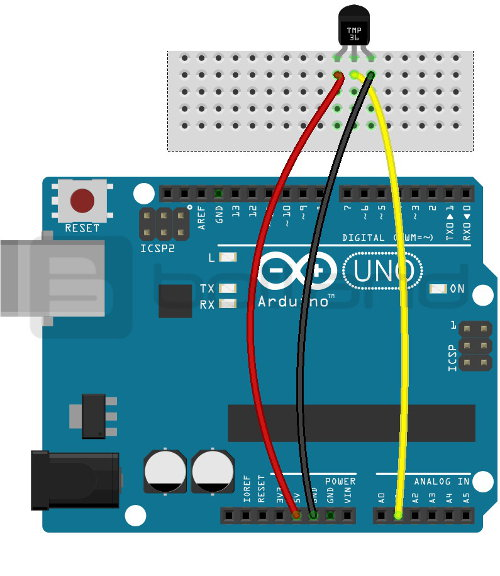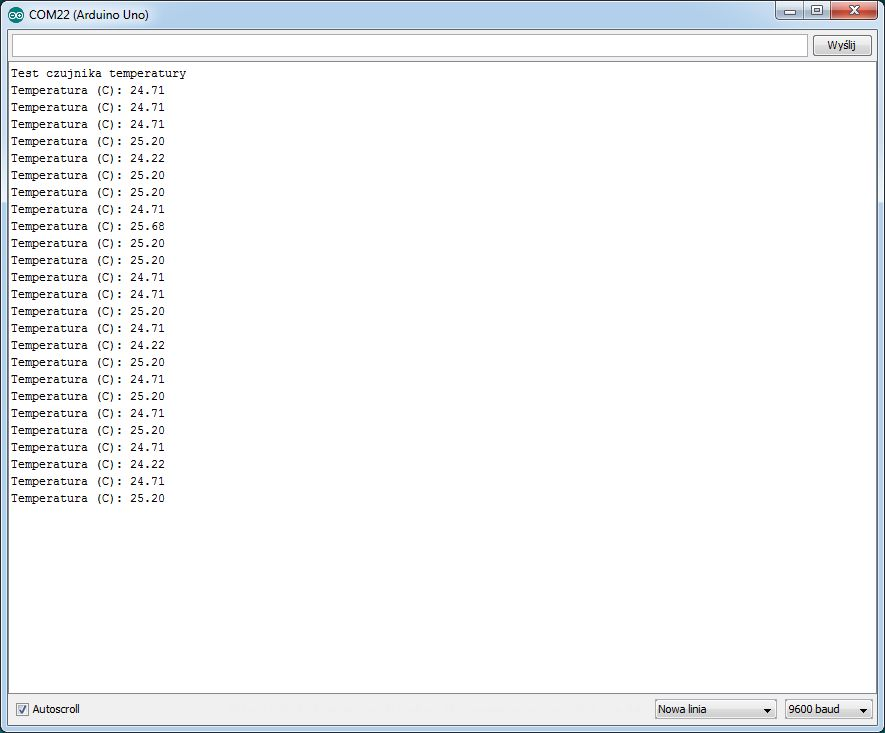# Odczyt temperatury z wykorzystaniem Arduino i czujnika TMP36GT9Z

## Read temperature with an Arduino and sensor TMP36GT9Z

A tutorial is a method of processing a temperature sensor TMP36GT9 using the Arduino module.

#### Connect the sensor with Arduino:

The sensor using the Arduino you should connect the layout as follows:

SensorPin Arduino
GNDGND
SignalA1
Vcc5 VThe connection scheme of sensor with the Arduino Uno.

#### Program for Arduino

In the example, we used the following code:

```int sensor = A1; //analog pin A1 is connected to the signal from the sensor
float VOLTS;
float TEMP;

void setup(){
Serial.begin(9600); //initialize serial monitor
Serial.println("Test temperature sensor");
}

void loop(){
VOLT = (reading * 5.0) / 1024.0; //conversion of the measured value for voltage in volts (for connection at 5 V)
TEMP = (VOLTS - 0.5) * 100; //conversion from voltage to temperature, the resolution of the sensor is 10 mV per degree, in addition, you should use an offset of 500 mV
Serial.print("Temperature (C): "); //display it on the monitor
Serial.println(TEMP);